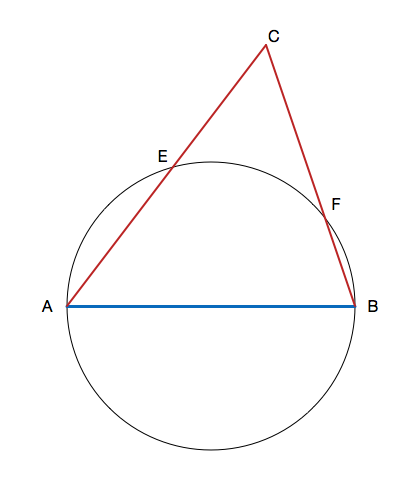# Skinny Triangle

Geometry Level 5Let $ABC$ be a triangle such that $AB=24$ is the diameter of of a circle $O$. Points $F$ and $E$ lie on the intersection of the circle and lines $BC$ and $AC$, respectively, such that $BF=1$ and $AE=3$. Find the perimeter of triangle $ABC$. Round your answer to the nearest integer.

This problem was created while accidentally misinterpreting an OMO problem. :D

×